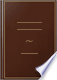Αναζήτηση Εικόνες Χάρτες Play YouTube Ειδήσεις Gmail Drive Περισσότερα »
Είσοδος
 Βιβλία Βιβλία 21 - 30 από 121 για RULE. Divide as in whole numbers, and from the right hand of the quotient point off....RULE. Divide as in whole numbers, and from the right hand of the quotient point off as many places for decimals as the decimal places in the dividend exceed those in the divisor.The Teachers' Assistant, Or, A System of Practical Arithmetic: Wherein the ... - Σελίδα 147
1817 - 198 σελίδες
Πλήρης προβολή - Σχετικά με αυτό το βιβλίο## The Complete Practical Arithmetician: Containing Several New and Useful ...

Thomas Keith - 1822 - 332 σελίδες
...in whole numbers, and from the right hand of the quotient point oft- so many figures for tlev-irjals as the decimal places in the dividend exceed those in the divisor ; but, if the quotient does not contain Midi a number of figures as is equal to the excess, the defect...## The New American Arithmetic, in the Coin of the United States, Denominated ...

John Lyman Newell - 1822 - 202 σελίδες
...must be equal to those in the dividend ; ie point off as many places for decimals in the quotient, as the decimal places in the dividend exceed those in the divisor. Q. When the decimals in the quotient are not so many as the rule requires, how do you proceed ? A....## The Arithmetical Catechism, Compiled from Various Authors, for the Use of ...

Henry Jackson - 1824 - 165 σελίδες
...sam€*as Simple Division. 2. Q. What «7/te rule for pointing in Division ? A. Point^t^T so.' many places as the decimal places .in the Dividend -exceed those in the Divisor. If the places of the Quotient be not so many as the rule requires. supply the defect by prefixing ciphers. 3. Q. When the decimal...## The Complete Practical Arithmetician: Containing Several New and Useful ...

Thomas Keith - 1825 - 332 σελίδες
...RULE I. Divide as in whole numbers, and from the right hand of the quotient point off so many figures for decimals as the decimal places in the dividend exceed those in the divisor; but, if the quotient does not contain such a number of figures as is equal to the excess, the defect...## A Course of Mathematics for the Use of Academies: As Well as ..., Τόμος 1

Charles Hutton - 1825
...DIVISION OF DECIMALS. DIVIDE as in whole numbers ; and point off in the quotient as many places f. >r decimals, as the decimal places in the dividend exceed those in the divisor.* » The reason of this Rule is evident; for, since the divisor multiplied by the quotient gives the...## Pike's System of Arithmetick Abridged: Designed to Facilitate the Study of ...

Nicolas Pike, Dudley Leavitt - 1826 - 200 σελίδες
...dividend ; therefore, divide as in whole numbers, and, from the right hand of the quotient, point off so many places for decimals, as the decimal places in the dividend exceed those in the divisor. 2. If the places of the quotient be not so many as the rule requires, supply the defect by prefixing...## The Youth's Assistant in Theoretick and Practical Arithmetick ...

Zadock Thompson - 1826 - 164 σελίδες
...DECIMALS. RULE.! — Divide as in whole numbers, and point oft' so many plates for decimals in the quotient as the decimal places in the dividend exceed those in the divisor. If there are not so many figures in the quotient as the number of decimals required, supply the defect...## The Common School Manual: A Regular and Connected Course of Elementary ...

...or short, of whole numbers. 2. Point off to the right of the quotient, as many places fpidecimals, as the decimal places in the dividend exceed those in the divisor. 3. If the places in the quotient fall short, supply the deficiency by prefixing cyphers. 4. When a...## The Improved Arithmetic: Newly Arranged and Clearly Illustrated, Both ...

Daniel Parker - 1828 - 348 σελίδες
...dividend. Therefore, divide as in whole numbers, and from the right-band of the quotient point off so many places for decimals, as the decimal places in the dividend exceed those in the divisor. 2. If the places in the quotient be not so many as the rule requires, supply the defect by prefixing...## The Federal Calculator: Or, A Concise System of Practical Arithmetic

William Slocomb - 1828 - 137 σελίδες
...dividend; therefore divide as in whole numbers, and from the right hand of the quotient, point off so many places for decimals, as the decimal places in the dividend exceed those in the divisor. o 2. If there be not so many places of decimals in the quotient as the rule requires, supply the deficit...